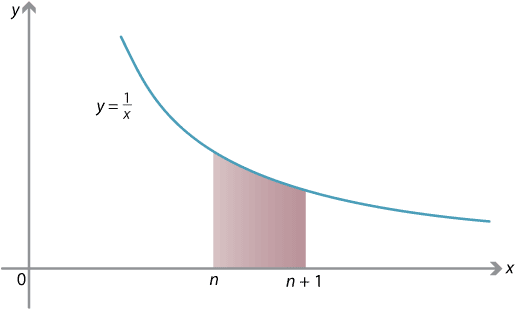### Striking it lucky with $$e$$

You're digging for gold on the goldfields. It's a plentiful gold field, and every time you dig, you obtain an amount of gold between 0 and 1 gram. The amount of gold you find each time is random and uniformly distributed. How many times do you expect to have to dig before you obtain a total of 1 gram of gold?

You might think that the answer is twice! Actually, the expected number of digs is $$e$$.

To put the question in pure mathematical terms, the amount of gold (in grams) you obtain each time you dig is a random variable uniformly distributed in $$[0,1]$$. Let $$X_1$$ be the amount you obtain the first time you dig, let $$X_2$$ be the amount the second time you dig and, in general, let $$X_n$$ be the amount of gold found the $$n$$th time you dig. So each $$X_n$$ is a random variable uniformly distributed in $$[0,1]$$.

The number of times you have to dig to obtain 1 gram is then the least $$n$$ such that $${X_1 + X_2 + \dotsb + X_n \geq 1}$$. Let this number be $$M$$. It is also a random variable:

$M = \min \bigl\{\, n : X_1 + X_2 + \dotsb + X_n \geq 1 \,\bigr\}.$

The number of times you expect to have to dig to obtain 1 gram of gold is the expected value $$E(M)$$ of $$M$$.

The probability that you obtain a full gram in your first dig is zero — you have to get a whole gram, and the chances of that are vanishingly small: $$\Pr(M=1)=0$$.

The probability you obtain a gram in two digs is $$\dfrac{1}{2}$$: you could get anywhere from 0 to 2 grams, and 1 is right in the middle.

In general, the probability that you obtain a gram on the $$n$$th dig, but not earlier, can be expressed as follows. It's the probability that you have less than a gram after $$n-1$$ digs, but are not still below a gram after $$n$$ digs:

\begin{align*} \Pr(M = n) &= \Pr(X_1 + \dotsb + X_{n-1} < 1 \text{ and } X_1 + \dotsb + X_n \geq 1) \\ &= \Pr(X_1 + \dotsb + X_{n-1} < 1) - \Pr(X_1 + \dotsb + X_n < 1). \end{align*}

As it turns out,4 the probability that you have less than a gram after $$n$$ digs is

$\Pr (X_1 + \dotsb + X_n < 1) = \dfrac{1}{n!}.$

Proving this requires some work with integration and probability distribution functions.

From this, we obtain

$\Pr(M=n) = \dfrac{1}{(n-1)!} - \dfrac{1}{n!}.$

Thus $$\Pr(M=1) = 0$$ and, for $$n>1$$, we can simplify to

\begin{align*} \Pr(M=n) &= \dfrac{1}{(n-1)!} \Bigl( 1 - \dfrac{1}{n} \Bigr)\\ &= \dfrac{1}{(n-1)!} \cdot \dfrac{n-1}{n}\\ &= \dfrac{1}{n} \cdot \dfrac{1}{(n-2)!}. \end{align*}

Knowing this probability, we see that the expected number of digs required is

\begin{align*} E(M) &= 1 \Pr(M=1) + 2 \Pr(M=2) + 3 \Pr (M=3) + 4 \Pr (M=4) + \dotsb \\ &= 1 \cdot 0 + 2 \cdot \dfrac{1}{2} \cdot \dfrac{1}{0!} + 3 \cdot \dfrac{1}{3} \cdot \dfrac{1}{1!} + 4 \cdot \dfrac{1}{4} \cdot \dfrac{1}{2!} + \dotsb \\ &= 1 + \dfrac{1}{1!} + \dfrac{1}{2!} + \dfrac{1}{3!} + \dotsb = e. \end{align*}

We have yet to hear of any goldfields with such a distribution of gold.

### Sandwiching $$e$$

By considering the integral

$\int_n^{n+1} \dfrac{1}{x} \; dx,$ where $$n$$ is positive, we will obtain another amazing formula for $$e$$.Detailed description

On the one hand, we can compute the integral exactly:

\begin{align*} \int_n^{n+1} \dfrac{1}{x} \; dx &= \bigl[ \ln x \bigr]_n^{n+1}\\ &= \ln (n+1) - \ln n\\ &= \ln \dfrac{n+1}{n}\\ &= \ln \Bigl( 1 + \dfrac{1}{n} \Bigr). \end{align*}

On the other hand, on the interval $$[n,n+1]$$, we see that the function $$\smash{\dfrac{1}{x}}$$ satisfies

$\dfrac{1}{n+1} \leq \dfrac{1}{x} \leq \dfrac{1}{n}.$

Using these inequalities to estimate the integral (see the module Integration for details), we have

$\dfrac{1}{n+1} \leq \int_n^{n+1} \dfrac{1}{x} \; dx = \ln \Bigl( 1 + \dfrac{1}{n} \Bigr) \leq \dfrac{1}{n}.$

Multiplying through by $$n$$ and using a logarithm law gives

$\dfrac{n}{n+1} \leq n \, \ln \Bigl( 1 + \dfrac{1}{n} \Bigr) = \ln \Bigl(1 + \dfrac{1}{n}\Bigr)^n \leq 1.$

The expression $$\ln (1 + \dfrac{1}{n})^n$$ is sandwiched between $$\dfrac{n}{n+1}$$ and 1. In fact, as $$n \to \infty$$, $$\dfrac{n}{n+1} \to 1$$ as well, and so we can deduce

$\lim_{n \to \infty} \ln \Bigl( 1 + \dfrac{1}{n} \Bigr)^n = 1.$

It follows that the limit of $$(1+\dfrac{1}{n})^n$$, as $$n \to \infty$$, is a number whose natural logarithm is 1, that is, $$e$$. We have our new formula for $$e$$:

$\lim_{n \to \infty} \Bigl( 1 + \dfrac{1}{n} \Bigr)^n = e.$

Exercise 21

Using a similar technique, prove that for any real $$x$$,

$\lim_{n \to \infty} \Bigl( 1 + \dfrac{x}{n} \Bigr)^n = e^x.$

Next page - History and applications